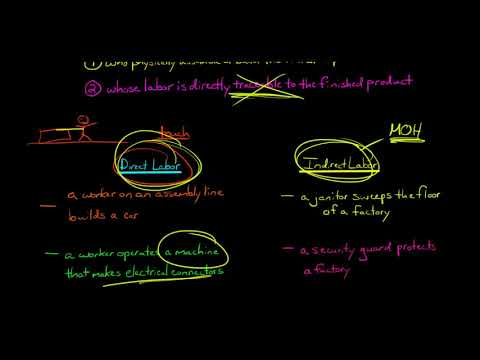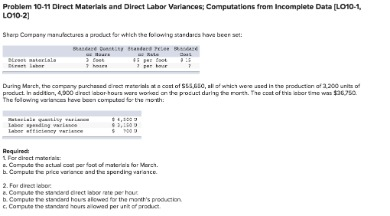# Direct labor efficiency variance calculatorAn adverse labor rate variance indicates higher labor costs incurred during a period compared with the standard. Direct labor costs are defined as a cost of labor that goes directly into the production or manufacturing of a good. These include the wages, taxes, and benefits paid to the employees that directly worked on the product over a time period.

• In this case, the actual rate per hour is \$7.50, the standard rate per hour is \$8.00, and the actual hour worked is 0.10 hours per box.
• To estimate how the combination of wages and hours affects total costs, compute the total direct labor variance.
• This is offset by a larger favorable direct labor rate variance of \$2,550.
• Enter the actual hours worked, the actual rate paid, and the standard rate pay into the calculator to determine the labor rate variance.

If the exam takes longer than expected, the doctor is not compensated for that extra time. Doctors know the standard and try to schedule accordingly so a variance does not exist. If anything, they try to produce a favorable variance by seeing more patients in a quicker time frame to maximize their compensation potential. If the outcome is unfavorable, the actual costs related to labor were more than the expected (standard) costs. If the outcome is favorable, the actual costs related to labor are less than the expected (standard) costs. The following equations summarize the calculations for direct labor cost variance.

With either of these formulas, the actual rate per hour refers to the actual rate of pay for workers to create one unit of product. The standard rate per hour is the expected rate of pay for workers to create one unit of product. The actual hours worked are the actual number of hours worked to create one unit of product. If there is no difference between the standard rate and the actual rate, the outcome will be zero, and no variance exists. Actual and standard quantities and rates for direct labor for the production of 1,000 units are given in the following table. Total actual and standard direct labor costs are calculated by multiplying number of hours by rate, and the results are shown in the last row of the first two columns.

## Total Direct Labor Variance

The difference column shows that 100 extra hours were used vs. what was expected (unfavorable). It also shows that the actual rate per hour was \$0.50 lower than standard cost (favorable). The total actual cost direct labor cost was \$1,550 lower than the standard cost, which is a favorable outcome. The difference in hours is multiplied by the standard price per hour, showing a \$1,000 unfavorable direct labor time variance. This is offset by a larger favorable direct labor rate variance of \$2,550. The net direct labor cost variance is still \$1,550 (favorable), but this additional analysis shows how the time and rate differences contributed to the overall variance.

• Direct Labor Rate Variance is the measure of difference between the actual cost of direct labor and the standard cost of direct labor utilized during a period.
• Each bottle has a standard labor cost of 1.5 hours at \$35.00 per hour.
• They pay a set rate for a physical exam, no matter how long it takes.
• Doctors know the standard and try to schedule accordingly so a variance does not exist.
• If the actual hours worked are less than the standard hours at the actual production output level, the variance will be a favorable variance.
• There are two components to a labor variance, the direct labor rate variance and the direct labor time variance.

Another element this company and others must consider is a direct labor time variance. The actual hours used can differ from the standard hours because of improved efficiencies in production, carelessness or inefficiencies in production, or poor estimation when creating the standard usage. A favorable labor rate variance suggests cost efficient employment of direct labor by the organization. A labor rate variance is a measure between the total amount paid for labor and the standard amount paid. Labor rate variance is the total difference between the total paid amount for a certain amount of labor and the standard amount that the labor usually commands. Managers can better address this situation if they have a breakdown of the variances between quantity and rate.

## Direct Labor Rate Variance

Specifically, knowing the amount and direction of the difference for each can help them take targeted measures forimprovement. Average acceleration is the object’s change in speed for a specific given time period.An unfavorable outcome means you paid workers more than anticipated. In this case, the actual rate per hour is \$7.50, the standard rate per hour is \$8.00, and the actual hour worked is 0.10 hours per box. This is a favorable outcome because the actual rate of pay was less than the standard rate of pay. In this case, the actual hours worked are 0.05 per box, the standard hours are 0.10 per box, and the standard rate per hour is \$8.00. This is a favorable outcome because the actual hours worked were less than the standard hours expected. According to the total direct labor variance, direct labor costs were \$1,200 lower than expected, a favorable variance.

If there is no difference between the actual hours worked and the standard hours, the outcome will be zero, and no variance exists. If the actual rate of pay per hour is less than the standard rate of pay per hour, the variance will be a favorable variance. If, however, the actual rate of pay per hour is greater than the standard rate of pay per hour, the variance will be unfavorable.

## Direct Labor Time Variance

With either of these formulas, the actual hours worked refers to the actual number of hours used at the actual production output. The standard rate per hour is the expected hourly rate paid to workers. The standard hours are the expected number of hours used at the actual production output.

In this case, two elements are contributing to the unfavorable outcome. Connie’s Candy paid \$1.50 per hour more for labor than expected and used 0.10 hours more than expected to make one box of candy. The same calculation is shown as follows using the outcomes of the direct labor rate and time variances. The total direct labor variance is also found by combining the direct labor rate variance and the direct labor time variance. By showing the total direct labor variance as the sum of the two components, management can better analyze the two variances and enhance decision-making.To compute the direct labor price variance, subtract the actual hours of direct labor at standard rate (\$43,200) from the actual cost of direct labor (\$46,800) to get a \$3,600 unfavorable variance. This result means the company incurs an additional \$3,600 in expense by paying its employees an average of \$13 per hour rather than \$12. To compute the direct labor quantity variance, subtract the standard cost of direct labor (\$48,000) from the actual hours of direct labor at standard rate (\$43,200). This math results in a favorable variance of \$4,800, indicating that the company saves \$4,800 in expenses because its employees work 400 fewer hours than expected. The difference between the standard cost of direct labor and the actual hours of direct labor at standard rate equals the direct labor quantity variance.

To estimate how the combination of wages and hours affects total costs, compute the total direct labor variance. As with direct materials, the price and quantity variances add up to the total direct labor variance. Each bottle has a standard labor cost of 1.5 hours at \$35.00 per hour. Calculate the labor rate variance, labor time variance, and total labor variance.

## Labor Rate Variance Formula

The combination of the two variances can produce one overall total direct labor cost variance. Because Band made 1,000 cases of books this year, employees should have worked 4,000 hours (1,000 cases x 4 hours per case). However, employees actually worked 3,600 hours, for which they were paid an average of \$13 per hour. Direct Labor Rate Variance is the measure of difference between the actual cost of direct labor and the standard cost of direct labor utilized during a period. A direct labor variance is caused by differences in either wage rates or hours worked. As with direct materials variances, you can use either formulas or a diagram to compute direct labor variances.

## Labor Costs in Service Industries

Doctors, for example, have a time allotment for a physical exam and base their fee on the expected time. Insurance companies pay doctors according to a set schedule, so they set the labor standard. They pay a set rate for a physical exam, no matter how long it takes.

## Employing diagrams to work out direct labor variances

If the actual hours worked are less than the standard hours at the actual production output level, the variance will be a favorable variance. A favorable outcome means you used fewer hours than anticipated to make the actual number of production units. If, however, the actual hours worked are greater than the standard hours at the actual production output level, the variance will be unfavorable. An unfavorable outcome means you used more hours than anticipated to make the actual number of production units.

The direct labor variance measures how efficiently the company uses labor as well as how effective it is at pricing labor. There are two components to a labor variance, the direct labor rate variance and the direct labor time variance. Enter the actual hours worked, the actual rate paid, and the standard rate pay into the calculator to determine the labor rate variance.